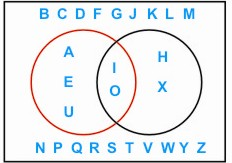HOME MATH DICTIONARY DOWNLOAD FEEDBACK DISCLAIMER
 Question: What is Venn Diagram ? Answer: A Venn diagram is a diagram where regions are used to represent the relation between two or more sets. It is a way of displaying how different sets of objects overlap. In the example shown here, the region inside the rectangle represents the letters of the alphabet, the region inside the red circle contains the vowels, and the region inside the black contains the letters with two lines of symmetry. The region common to the two circles then contains the vowels with two lines of symmetry. Venn diagrams are named after John Venn (1834 - 1923) a Cambridge mathematician.